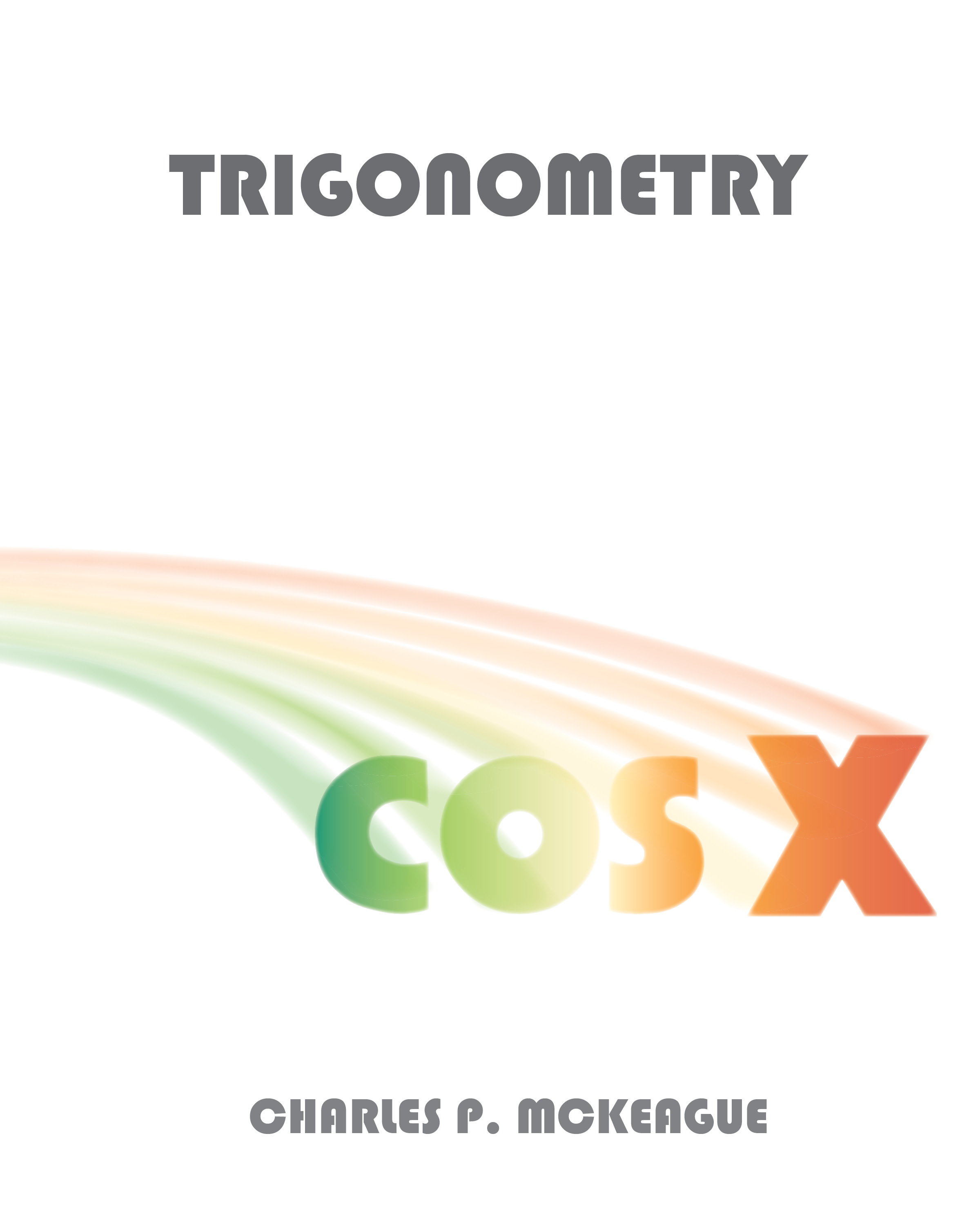# Trigonometry

Author:

Charles P. McKeague

Publisher:

XYZ TextbooksAn All-Access Pass gives you 1 full year of complete access to ALL of our online content, including all of our eBooks, MathTV videos, and online worksheets. An All Access Pass is included with every new XYZ Textbook.

$45 per year Paperback and eBook • ISBN: 978-1-630982-69-0$ 58
##### Product Information
Trigonometry

This trigonometry book is a standard right triangle approach to trigonometry. Each section is written so that it can be discussed in a 45 to 50 minute class session. The text covers all the material usually taught in trigonometry.

The emphasis of the book is on understanding the definitions and principles of trigonometry and their application to problem solving. However, when memorization is necessary, I say so.

Identities are introduced early in the book in Chapter 1. They are re­ viewed often and are then covered in more detail in Chapter 5. Also, exact values of the trigonometric functions are emphasized throughout the book. There are numerous calculator notes placed throughout the text that indicate how the examples and problems could be solved using a calculator.

ORGANIZATION OF THE TEXT The book begins with a preface to the student explaining what study habits are necessary to ensure success in mathematics.

The rest of the book is divided into chapters. Each chapter is organized as follows:

Chapter Introduction Each chapter begins with a preface that explains in a very general way what the student can expect to find in the chapter and some of the applications associated with topics in the chapter.

Sections Following the preface to each chapter, the body of the chapter is divided into sections. Each section contains explanations and examples. The explanations are as simple and intuitive as possible. The examples are chosen to clarify the explanations and preview the problems in the problem sets.

Problem Sets Following each section of the text is a problem set. There are five main ideas incorporated into each of the problem sets.

• DrillThere are enough problems in each problem set to ensure proficiency with the material once students have completed all the odd-numbered problems.

• Progressive DifficultyThe problems increase in difficulty as the problem set progresses.

• Odd-Even SimilaritiesEach pair of consecutive problems is similar. The answers to the odd-numbered problems are listed in the back of the book, and in many cases a short note or partial solution accompanies the answers to these problems. This gives students a chance to check their work and then try a similar problem.

• Application ProblemsWhenever possible, I have included a few application problems toward the end of each problem set. My experience is that students are always curious about how the trigonometry they are learning can be applied. They are also much more likely to put some time and effort into trying application problems if there are not an overwhelming number of them to work.

• Review ProblemsStarting with Chapter 2, each problem set ends with a few review problems. Generally, these review problems cover material that will be used in the next section. I find that assigning the review problems helps prepare my students for the next day's lecture and makes reviewing part of their daily routine.

Chapter Summaries  Following the last problem set in each chapter is a chapter summary. Each chapter summary lists all the properties and definitions found in the chapter. In the margin of each chapter summary, next to most of the topics being summarized, is an example that illustrates the kind of problem associated with that topic.

Chapter Tests Each chapter ends with a chapter test. These tests are designed to give students an idea of how well they have mastered the material in the chapter. All answers for these chapter tests are included in the back of the book.

## Instructor Resources

Instructor's Resource Manual- Contains two tests for each chapter of the book, and answers to all the problems in the text.

Complete Solutions Manual- Available online, this manual contains complete solutions to all the exercises in the problem sets.

Printed Test Bank- Instructors can choose from a bank of pre-created tests; two test for each chapter of the book. These are provided in an editable format so that they can be tailored to your teaching style and testing preferences.

PowerPoint Presentations- Presentations that can be opened in PowerPoint, Apple Keynote or Google Slides have been provided as a template for classroom lectures. Instructors can add examples that they want to use in class or use these to supplement their presentation of the material.

### for Trigonometry

##### Supplements

MathTV.com- Every example in every XYZ Textbook is worked on video by multiple video tutors. Students benefit from seeing multiple approaches and gain confidence in learning from their peers. You can find the MathTV videos in the XYZ eBook.

XYZ eBook- Access the eBook for this course as well as any other XYZ Textbook. Use the My Bookshelf feature under MY ACCOUNT to easily access your eBook.